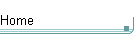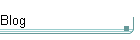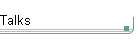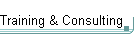GotW #55On theblogNovember 4: Other Concurrency Sessions at PDC November 3 October 26: Hoare on Testing October 23This is the original GotW problem and solution substantially as posted to Usenet. See the book More Exceptional C++ (Addison-Wesley, 2002) for the most current solution to this GotW issue. The solutions in the book have been revised and expanded since their initial appearance in GotW. The book versions also incorporate corrections, new material, and conformance to the final ANSI/ISO C++ standard.

## Equivalent Code? Difficulty: 5 / 10

Can subtle code differences really matter, especially in something as simple as postincrementing a function parameter? This issue explores an interesting interaction that becomes important in STL-style code.### Problem

#### JG Question

1. Describe what the following code does:

```    //  Example 1
//
f( a++ );```

Be as complete as you can about all possibilities.

#### Guru Questions

2. What is the difference, if any, between the following two code fragments?

```    //  Example 2(a)
//
f( a++ );```
```    //  Example 2(b)
//
f( a );
a++;```

3. In Question #2, make the simplifying assumption that f() is a function that takes its argument by value, and that a is an object of class type that has an operator++(int) with natural semantics. Now what is the difference, if any, between Example 2(a) and Example 2(b)?### Solution

1. Describe what the following code does:

```    //  Example 1
//
f( a++ );```

Be as complete as you can about all possibilities.

A comprehensive list would be daunting, but here are the main possibilities.

First, f could be any of the following:

1. A macro. In this case the statement could mean just about anything, and "a++" could be evaluated many times or not at all. For example:

```    #define f(x) x                      // once
#define f(x) (x,x,x,x,x,x,x,x,x)    // 9 times
#define f(x)                        // not at all```

Guideline: Avoid using macros. They usually make code more difficult to understand, and therefore more troublesome to maintain.

2. A function. In this case, first "a++" is evaluated and then the result is passed to the function as its parameter. Normally, postincrement returns the previous value of a in the form of a temporary object, so f() could take its parameter either by value or by reference to const, but not by reference to non-const because a reference to non-const cannot be bound to a temporary object.

3. An object. In this case, f() would be a functor, that is, an object for which operator()() is defined. Again, if postincrement returns the previous value of a (as postincrement always should) then f's operator()() could take its parameter either by value or by reference to const.

4. A type name. In this case, the statement first evaluates "a++" and uses the result of that expression to initialize a temporary object of type f.

Next, a could be:

1. A macro. In this case, again, a could mean just about anything.

2. An object (possibly of a built-in type). In this case, it must be a type for which a suitable operator++(int) postincrement operator is defined.

Normally, postincrement should be implemented in terms of preincrement and should return the previous value of a:

```    // Canonical form of postincrement:
T T::operator++(int)
{
T old( *this ); // remember our original value
++*this;        // always implement postincrement
//  in terms of preincrement
return old;     // return our original value
}```

When you overload an operator, of course, you do have the option of changing its normal semantics to do "something unusual." For example, the following is likely to break the Example 1 code for most kinds of f, assuming a is of type A:

`    void A::operator++(int) // doesn't return anything`

Don't do that. Instead, follow this sound advice:

Guideline: Always preserve natural semantics for overloaded operators. "Do as the ints do," that is, follow the semantics of the builtin types.

3. A value, such as an address. For example, a could be the name of an array, or it could be a pointer.

For the remaining questions, I will make the simplifying assumptions that:

- f() is not a macro; and

- a is an object with natural postincrement semantics.

2. What is the difference, if any, between the following two code fragments?

```    //  Example 2(a)
//
f( a++ );```

This performs the steps:

1. a++: Increment a and return the old value.

2. f(): Execute f(), passing it the old value of a.

Example 2(a) ensures that the postincrement is performed, and therefore a gets its new value, before f() executes. As noted above, f() could still be a function, a functor, or a type name which leads to a constructor call.

Some coding standards state that operations like ++ should always appear on separate lines, on the grounds that it can be dangerous to perform multiple operations like ++ in the same statement because of sequence points (more about this in GotW #56). Instead, such coding standards would recommend:

```    //  Example 2(b)
//
f( a );
a++;```

This performs the steps:

1. f(): Execute f(), passing it the old value of a.

2. a++: Increment a and return the old value, which is then ignored.

In both cases, f() gets the old value of a. "So what's the big difference?" you may ask. Well, Example 2(b) will not always have the same effect as that in Example 2(a), because Example 2(b) ensures that the postincrement is performed, and therefore a gets its new value, after f() executes. This has two major consequences: First, in the case where f() emits an exception, Example 2(a) ensures that a++ and all of its side effects have already been completed successfully, whereas Example 2(b) ensures that a++ has not been performed and none of its side effects have occurred.

Second, even in non-exceptional cases, if f() and a.operator++(int) have visible side effects, the order in which they are executed can matter. But, more specifically, consider what happens if f() has a side effect that affects the state of a itself: That's neither farfetched nor unlikely, and it can happen even if f() doesn't and can't directly change a, as I'll illustrate with an example:

3. In Question #2, make the simplifying assumption that f() is a function that takes its argument by value, and that a is an object of class type that has an operator++(int) with natural semantics. Now what is the difference, if any, between Example 2(a) and Example 2(b)?

The difference is that, for perfectly normal C++ code, Example 2(a) can be legal when Example 2(b) is not. Specifically, consider what happens when we replace f with list::erase(), and a with list::iterator. Now the first form is valid:

```    //  Example 3(a)
//
//  l is a list<int>
//  i is a valid non-end iterator into l```
`    l.erase( i++ ); // OK`

But the second form is not:

```    //  Example 3(b)
//
//  l is a list<int>
//  i is a valid non-end iterator into l```
```    l.erase( i );
i++;            // error, i is not a valid iterator```

The reason that Example 3(b) is incorrect is that the call to "l.erase( i )" invalidates i, and therefore you can no longer call operator++ on i afterwards.

#### Scissors, Traffic, and Iterators

Warning: Some programmers routinely write code like Example 3(b), perhaps because of coding guidelines that have a blanket policy of discouraging operations like ++ in function call statements.

If you're one of the programmers who writes code like Example 3(b), you may even be routinely getting away with it (and not realizing the danger) just because it happens to work on the current version of your compiler and library. But be warned: Code like Example 3(b) is not portable, it is not sanctioned by the Standard, and it's likely to turn and bite you when you port to another compiler platform or even just upgrade the one you're working on today. When it does bite, it will bite hard, because "using-an-invalid-iterator" bugs can be very difficult to find (unless you have the joy of working with a good checked library implementation during debugging -- but if you're in this situation you must not be using a checked implementation or else it would already have warned you about this!).

Some mothers (who are also software engineers) give the following three pieces of good advice, and we should always strive to follow them for our own good:

1. Don't run with scissors.

2. Don't play in traffic.

3. Don't use invalid iterators.

Next time: With the exception of examples like the above, we'll see why it's still a good idea in general to avoid writing operations like ++ in function calls.